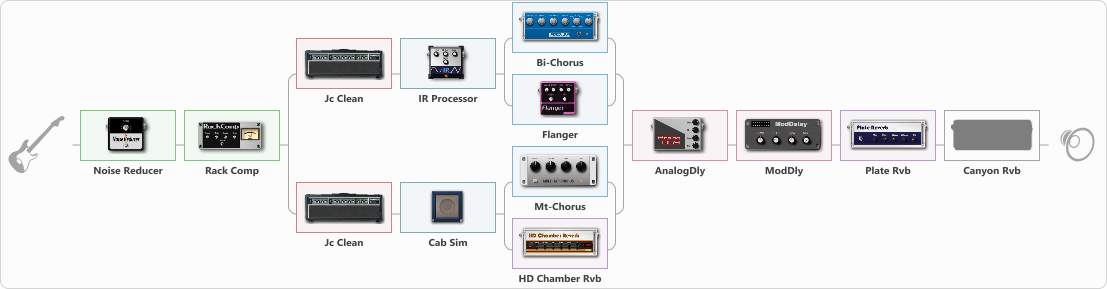# Dimension Truss

Discussion in 'ToneLib-GFX presets' started by truss van halen, Oct 12, 2020.

1. ### truss van halenWell-Known Member

Dimension Truss

Preset name: Dimension Truss

Effects chain:Effect: "Noise Reducer" (Dynamics / Filter), active - "yes"
{
"Sens" = 50
"Mode" = Soft
}

Effect: "Rack Comp" (Dynamics / Filter), active - "yes"
{
"Threshold (dB)" = -23
"Ratio" = 5
"Attack" = Slow
"Release (ms)" = 250
"Knee" = 34
"Level (dB)" = 7
}

Effect: "Splitter" (Dynamics / Filter), active - "yes"
{
"A-Bypass" = Off
"A-Pan" = -30
"A-Level" = 60
"B-Bypass" = Off
"B-Pan" = 30
"B-Level" = 60

'A' branch:
{

Effect: "Jc Clean" (Amp simulators), active - "yes"
{
"Gain" = 30
"Bass" = 58
"Middle" = 83
"Treble" = 36
"Presence" = 65
"Master" = 61
"Level (dB)" = 4
}

Effect: "IR Processor" (Cabinets), active - "yes"
{
"IR" = Cab IR Sample4
"Low Cut (Hz)" = 0
"Hi Cut (kHz)" = 20.0
"Mix" = 100
"Level (dB)" = 4
}

Effect: "Splitter" (Dynamics / Filter), active - "yes"
{
"A-Bypass" = Off
"A-Pan" = -100
"A-Level" = 40
"B-Bypass" = Off
"B-Pan" = 0
"B-Level" = 79

'A' branch:
{

Effect: "Bi-Chorus" (Modulation / Sfx), active - "yes"
{
"Speed A" = 1.8
"Speed B" = 3.6
"Depth" = 71
"Reso" = 30
"Mode" = OppPhase
"Mix" = 50
}
}
'B' branch:
{

Effect: "Flanger" (Modulation / Sfx), active - "yes"
{
"Speed" = 0.4
"Depth" = 82
"Reso" = 52
"Center" = 6.4
"Offset" = 5.0
"Mix" = 65
"Reset" = Off
}
}
}
}
'B' branch:
{

Effect: "Jc Clean" (Amp simulators), active - "yes"
{
"Gain" = 30
"Bass" = 55
"Middle" = 60
"Treble" = 23
"Presence" = 65
"Master" = 81
"Level (dB)" = 4
}

Effect: "Cab Sim" (Cabinets), active - "yes"
{
"Model" = 4x12" Greenback
"Level (dB)" = 4
}

Effect: "Splitter" (Dynamics / Filter), active - "yes"
{
"A-Bypass" = Off
"A-Pan" = -80
"A-Level" = 55
"B-Bypass" = Off
"B-Pan" = 67
"B-Level" = 83

'A' branch:
{

Effect: "Mt-Chorus" (Modulation / Sfx), active - "yes"
{
"Speed" = 1.5
"Depth" = 92
"Time" = 7.7
"Mix" = 100
}
}
'B' branch:
{

Effect: "HD Chamber Rvb" (Reverberation), active - "yes"
{
"Time" = 9.0
"PreLPF" = 79
"PreDelay" = 60
"HiDamp" = 21
"LoGain" = -2.1
"Mix" = 73
}
}
}
}
}

Effect: "AnalogDly" (Delay), active - "yes"
{
"Time" = Note sync, 1/16 Dotted
"Feedback" = 24
"Tone" = 50
"Mix" = 50
}

Effect: "ModDly" (Delay), active - "yes"
{
"Time" = Note sync, 1/4
"Feedback" = 65
"Tone" = 50
"Speed" = 1.3
"Mix" = 39
}

Effect: "Plate Rvb" (Reverberation), active - "yes"
{
"Time" = 2.9
"PreDelay" = 16
"LoDamp" = 17
"HiDamp" = 25
"Mix" = 42
}

Effect: "Canyon Rvb" (Reverberation), active - "no"
{
"Time" = 6.1
"PreDelay" = 42
"LoDamp" = 0
"HiDamp" = 32
"Mix" = 39
}

Note: You will need to download and install the ToneLib-GFX software to use the preset.

File size:
4.8 KB
Views:
2,577